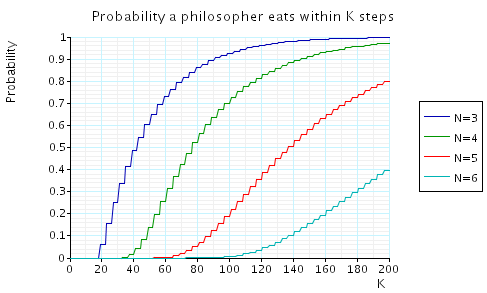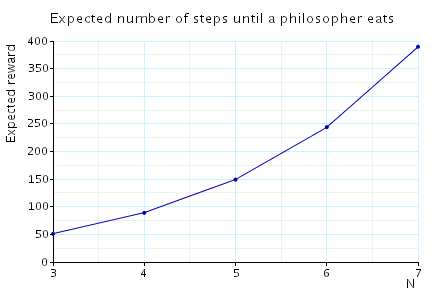# Randomised Dining Philosophers

(Lehmann and Rabin)

### Introduction

This case study is based on Lehmann and Rabin's [LR81] randomised solution to the well known dining philosophers problem. Note that this protocol has also been studies in [PZ86]. We consider both the original algorithm and the version presented in [DFP04] which removes the need to consider 'fairness' assumptions on the scheduling mechanism.

### Original Algorithm

This algorithm is Lehmann and Rabin's [LR81] solution to the well known dining philosophers problem. The model is represented as an MDP. We let N denotes the number of philosophers. By way of example, the PRISM source code for the original algorithm in the case where N = 3 is given below.

```// randomized dining philosophers [LR81]
// dxp/gxn 12/12/99
// atomic formulae
// left fork free and right fork free resp.
formula lfree = (p2>=0&p2<=4)|p2=6|p2=10;
formula rfree = (p3>=0&p3<=3)|p3=5|p3=7|p3=11;

module phil1

p1: [0..11];

[] p1=0 -> (p1'=0); // stay thinking
[] p1=0 -> (p1'=1); // trying
[] p1=1 -> 0.5 : (p1'=2) + 0.5 : (p1'=3); // draw randomly
[] p1=2 &  lfree -> (p1'=4); // pick up left
[] p1=2 &  !lfree -> (p1'=2); // left not free
[] p1=3 &  rfree -> (p1'=5); // pick up right
[] p1=3 &  !rfree -> (p1'=3); // right not free
[] p1=4 &  rfree -> (p1'=8); // pick up right (got left)
[] p1=4 & !rfree -> (p1'=6); // right not free (got left)
[] p1=5 &  lfree -> (p1'=8); // pick up left (got right)
[] p1=5 & !lfree -> (p1'=7); // left not free (got right)
[] p1=6  -> (p1'=1); // put down left
[] p1=7  -> (p1'=1); // put down right
[] p1=8  -> (p1'=9); // move to eating (got forks)
[] p1=9  -> (p1'=10); // finished eating and put down left
[] p1=9  -> (p1'=11); // finished eating and put down right
[] p1=10 -> (p1'=0); // put down right and return to think
[] p1=11 -> (p1'=0); // put down left and return to think

endmodule

// construct further modules through renaming
module phil2 = phil1 [ p1=p2, p2=p3, p3=p1 ] endmodule
module phil3 = phil1 [ p1=p3, p2=p1, p3=p2 ] endmodule

// labels
label "hungry" = ((p1>0)&(p1<8))|((p2>0)&(p2<8))|((p3>0)&(p3<8));
label "eat" = ((p1>=8)&(p1<=9))|((p2>=8)&(p2<=9))|((p3>=8)&(p3<=9));

```

### Model Statistics

The table below shows statistics for the MDPs we have built for different values of the constants N and K. The tables include:

• the number of states and transitions in the MDP representing the model;
• both the number of nodes and leaves of the MTBDD which represents the model;
• the construction time which equals the time taken for the system description to be parsed and converted to the MTBDD representing it, and the time to perform reachability, identify the non-reachable states and filter the MTBDD accordingly;
• the number of iterations required to find the reachable states (which is performed via a BDD based fixpoint algorithm).
 N: Model: MTBDD: Construction: States: Transitions: Nodes: Leaves: Time (s): Iter.s: 3 956 3,696 910 3 0.124 18 4 9,440 48,656 1,958 3 0.141 24 5 93,068 599,600 3,335 3 0.311 30 6 917,424 7,092,696 5,008 3 0.782 36 7 9,043,420 81,568,144 6,968 3 1.568 42 8 89,144,512 918,913,056 9,215 3 2.942 48 9 878,732,012 10190,342,448 11,749 3 5.025 54 10 8,662,001,936 111,611,282,280 14,570 3 7.532 60 15 8.06e+15 1.56e+17 32,980 3 37.85 90 20 7.50e+19 1.93e+21 58,565 3 105.3 120 25 6.98e+24 2.24e+26 91,325 3 1,000 150 30 6.45e+29 2.51e+31 13,1260 3 2,719 180

### Model Checking

When model checking, we have the following requirement:

• Scheduler-Liveness: "For each time t and any philosopher Pi, there exists a time t' > t in which Pi makes a move."

This corresponds to the conditions on the adversary/scheduler which we impose by model checking against only fair adversaries.

We have model checked the following liveness property: 'If a philosopher is hungry, then eventually some philosopher eats'.

```// liveness (if a philosopher is hungry then eventually some philosopher eats)
"hungry" => P>=1 [ true U "eat"]```
Model checking times:

 N: Precomputation: Main Algorithm: Time (s): Iterations: Time (s): Iterations: 3 0.01 6 - - 4 0.03 6 - - 5 0.07 6 - - 6 0.08 6 - - 7 0.13 6 - - 8 0.17 6 - - 9 0.23 6 - - 10 0.29 6 - - 15 0.73 6 - - 20 1.36 6 - - 25 2.20 6 - - 30 5.22 6 - -

Conclusion: the property holds in all states

### Algorithm Without Fairness Assumptions

This algorithm is the version of Lehmann and Rabin's dining philosopher algorithm presented in [DFP04] and does not require any fairness assumptions. The model is represented as an MDP. We let N denotes the number of philosophers. By way of example, the PRISM source code for the algorithm of [DFP04] in the case of 3 philosophers is given below. The difference between this algorithm and that originally presented by Lehmann and Rabin is that the philosophers cannot be scheduled if their next transition is a 'loop', i.e. such transitions are removed from the model.

```// randomized dining philosophers [LR81]
// dxp/gxn 23/01/02

// model which does not require fairness
// remove the possibility of loops:
// (1) cannot stay in thinking
// (2) if first fork not free then cannot move (another philosopher must more)

mdp

// atomic formulae
// left fork free and right fork free resp.
formula lfree = (p2>=0&p2<=4)|p2=6|p2=10;
formula rfree = (p3>=0&p3<=3)|p3=5|p3=7|p3=11;

module phil1

p1: [0..11];

[] p1=0 -> (p1'=1); // trying
[] p1=1 -> 0.5 : (p1'=2) + 0.5 : (p1'=3); // draw randomly
[] p1=2 &  lfree -> (p1'=4); // pick up left
[] p1=3 &  rfree -> (p1'=5); // pick up right
[] p1=4 &  rfree -> (p1'=8); // pick up right (got left)
[] p1=4 & !rfree -> (p1'=6); // right not free (got left)
[] p1=5 &  lfree -> (p1'=8); // pick up left (got right)
[] p1=5 & !lfree -> (p1'=7); // left not free (got right)
[] p1=6  -> (p1'=1); // put down left
[] p1=7  -> (p1'=1); // put down right
[] p1=8  -> (p1'=9); // move to eating (got forks)
[] p1=9  -> (p1'=10); // finished eating and put down left
[] p1=9  -> (p1'=11); // finished eating and put down right
[] p1=10 -> (p1'=0); // put down right and return to think
[] p1=11 -> (p1'=0); // put down left and return to think

endmodule

// construct further modules through renaming
module phil2 = phil1 [ p1=p2, p2=p3, p3=p1 ] endmodule
module phil3 = phil1 [ p1=p3, p2=p1, p3=p2 ] endmodule

// rewards (number of steps)
rewards "num_steps"
[] true : 1;
endrewards
// labels
label "hungry" = ((p1>0)&(p1<8))|((p2>0)&(p2<8))|((p3>0)&(p3<8));
label "eat" = ((p1>=8)&(p1<=9))|((p2>=8)&(p2<=9))|((p3>=8)&(p3<=9));

```

### Model Statistics

The table below shows statistics for the MDPs we have built for different values of the constants N and K. The tables include:

• the number of states and transitions in the MDP representing the model;
• both the number of nodes and leaves of the MTBDD which represents the model;
• the construction time which equals the time taken for the system description to be parsed and converted to the MTBDD representing it, and the time to perform reachability, identify the non-reachable states and filter the MTBDD accordingly;
• the number of iterations required to find the reachable states (which is performed via a BDD based fixpoint algorithm).
 N: Model: MTBDD: Construction: States: Transitions: Nodes: Leaves: Time (s): Iter.s: 3 956 3,048 765 3 0.065 18 4 9,440 40,120 1,650 3 0.121 24 5 93,068 494,420 2,854 3 0.259 30 6 917,424 5,848,524 4,339 3 0.612 36 7 9,043,420 67,259,808 6,101 3 1.18 42 8 89,144,512 757,721,264 8,150 3 2.31 48 9 878,732,012 8,402,796,252 10,486 3 3.74 54 10 8,662,001,936 92,032,909,540 13,109 3 5.83 60

### Model Checking

We have model checked the following properties:

```const int K; // discrete time bound

// liveness (if a philosopher is hungry then eventually some philosopher eats)
"hungry" => P>=1 [ true U "eat"]

// bounded waiting (minimum probability, from a state where someone is hungry, that a philosopher will eat within K steps)
Pmin=?[true U<=K "eat" {"hungry"}{min}]

// expected time (from a state where someone is hungry the maximum exapected number of steps until a philosopher eats)
Rmax=?[F "eat" {"hungry"}{max}]
```